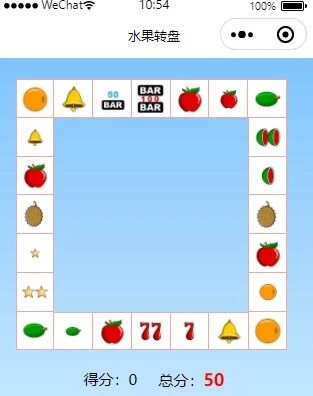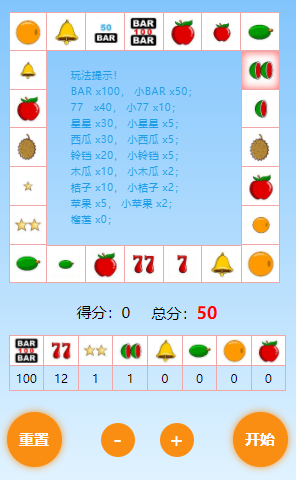# 置顶微信小程序实现水果老虎机游戏精选热门

## 游戏介绍

BAR x100，小BAR x50；77  x40， 小77 x10；星星 x30， 小星星 x5；西瓜 x30， 小西瓜 x5；铃铛 x20， 小铃铛 x5；木瓜 x10， 小木瓜 x2； 桔子 x10， 小桔子 x2；苹果 x5， 小苹果 x2；榴莲 x0；

## 使用技术

• CSS：盒子模型，定位，浮动，CSS3的Flex弹性盒模型，图片，CSS3选择器...
• JS：json，sort，数组，定时器，随机数，设计概率...

### 水果机转盘界面设计

```.ul{
position: relative;
height: 650rpx;
width: 650rpx;
margin-left: auto;
margin-right: auto;
}
.li{
width: 14%;
height:14%;
list-style: none;
text-align: center;
line-height: 90rpx;
position: absolute;
background-size: contain;
border: 1rpx solid #f1a7a7;
background-color: white;
}
```

​现在基本的样子就出来了​最后整体布局如下：```startPlay() {
let fruits = that.data.fruits; //转盘数组
let flen = fruits.length;
let fruitsBet = this.data.fruitsBet; //投注数组
let step = flen * 2 + parseInt(Math.random() * 35); //随机数，转盘焦点定位
let index = that.data.activeIndex; //转盘数组下标

function start() {
that.setData({
activeLeft: fruits[index].left,
activeTop: fruits[index].top
})
step--;
if (step == 0) {
clearTimeout(that.data.timerID);
index++;
if (index == fruits.length) {
index = 0;
}
that.setData({
activeIndex: index
})
that.data.timerID = setTimeout(start, 1800 / step);
}
start(step)

}
```

activeLeft和activeTop是转灯的定位，fruits是存放转盘图片的数组，每个图片的位置都写在了数组里面，这样通过每次运行index来获取每个图片的位置，赋值给转灯达到转动的效果。step是随机产生的数字让转灯最少运行两圈。

baseNum: 5, //基数，越大投中几率越低

bigMin: 3, //出现大号的基数

```let newFruit = fruitsBet.slice().sort(that.compareDown("bet","score")).slice(0,5); //投注倒序​
//倒序
compareDown(bet,score) {
return function (a, b) {
var bet1 = a[bet] * a[score];
var bet2 = b[bet] * b[score];
return bet1 - bet2;
}
},
```

​抽出最低的五个押注，然后在这五个里面产生随机数。这还没完，因为这五个里面还有大小的区分，就像前面说的那样通过大号基数设置大号出现的概率。

​​

```if (runNum % baseNum == 0) {
step = flen * 2 + parseInt(Math.random() * 35 );
} else {
let descid = 0;
let bigMin = that.data.bigMin;
let seleID = 0;
let seleScore = 0;
if (runNum % bigMin == 0) {
descid = parseInt(Math.random() * newFruitMbIg.length + 1) - 1;
seleID = newFruitMbIg[descid].index;
seleScore = newFruitMbIg[descid].score;
} else {
descid = parseInt(Math.random() * newFruitMin.length + 1) - 1;
seleID = newFruitMin[descid].index;
seleScore = newFruitMin[descid].score;
}
fruits.forEach((item, i) => {
if (item.index == seleID && item.score == seleScore) {
if (i < index) {
step = flen * 3 - (index - i) + 1;
} else {
step = flen * 2 + i - index + 1;
}
}
})
}
```

​通过这样一通设置后整个水果老虎机基本完成。欢迎大家扫码体验，有问题可以做小程序内反馈。